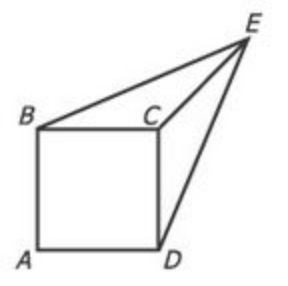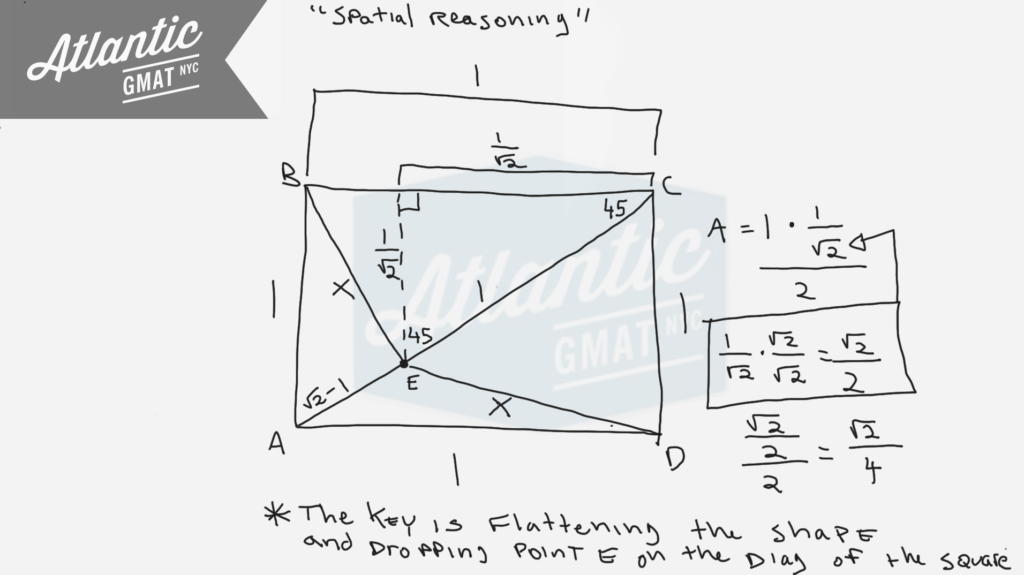Private GMAT Tutoring in NYC & OnlineIn the figure above, each side of square ABCD has length 1, the length of line segment CE is 1, and the length of line segment BE is equal to the length of line segment DE. What is the area of the triangular region BCE?

(A) 1/3
(B) √2/4
(C) 1/2
(D) √2/2
(E) 3/4

This is a tough geometry question from the GMAT prep tests (I think it's from exam pack 1, tests 3/4). The first time I saw this one I made the mistake of assuming a 3 dimensional figure. The question is much easier once you put the shape in 2 dimensions dropping point E onto the diagonal of square ABCD. At that point it becomes a set of somewhat straightforward inferences. There are two important inferences to make:

1. For a square always define the diagonal. It comes up a ton on GMAT geometry questions.
2. If you're looking for the area of a triangle you'll need a height. If not dealing with a right triangle go ahead and always draw an altitude/height.

Once you've done those two things finding the area of the triangular region BCE is a piece of cake.# CONTACT

Atlantic GMAT

405 East 51st St.

NY, NY 10022

(347) 669-3545

info@AtlanticGMAT.com